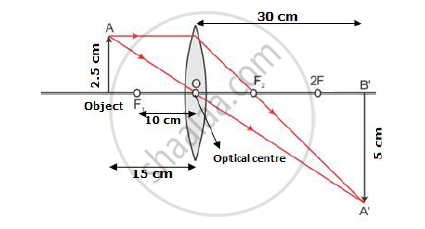An object of height 2.5 cm is placed at a distance of 15 cm from the optical centre 'O' of a convex lens of focal length 10 cm. Draw a ray diagram to find the position and size of the image formed. - Science

An object of height 2.5 cm is placed at a distance of 15 cm from the optical centre 'O' of a convex lens of focal length 10 cm. Draw a ray diagram to find the position and size of the image formed. Mark optical 'O', principal focus F and height of the image on the diagram.

Solution

Ray diagram:Concept: Convex Lens
Is there an error in this question or solution?